# Kernel Regression

## Motivation

For observed pairs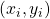,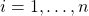, the relationship between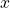and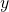can be defined generally as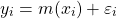where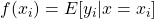and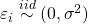. If we are unsure about the form of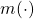, our objective may be to estimatewithout making too many assumptions about its shape. In other words, we aim to “let the data speak for itself”.

Simulated scatterplot of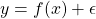. Here,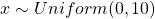and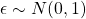. The true function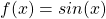is displayed in green.

Non-parametric approaches require only thatbe smooth and continuous. These assumptions are far less restrictive than alternative parametric approaches, thereby increasing the number of potential fits and providing additional flexibility. This makes non-parametric models particularly appealing when prior knowledge about‘s functional form is limited.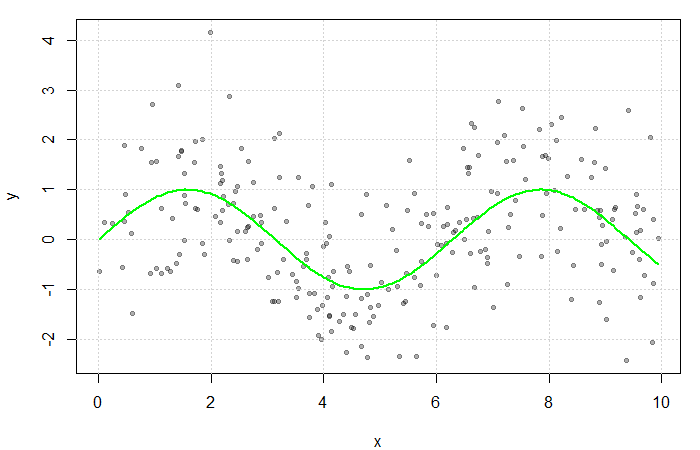## Estimating the Regression Function

If multiple values ofwere observed at each,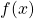could be estimated by averaging the value of the response at each. However, sinceis often continuous, it can take on a wide range of values making this quite rare. Instead, a neighbourhood ofis considered.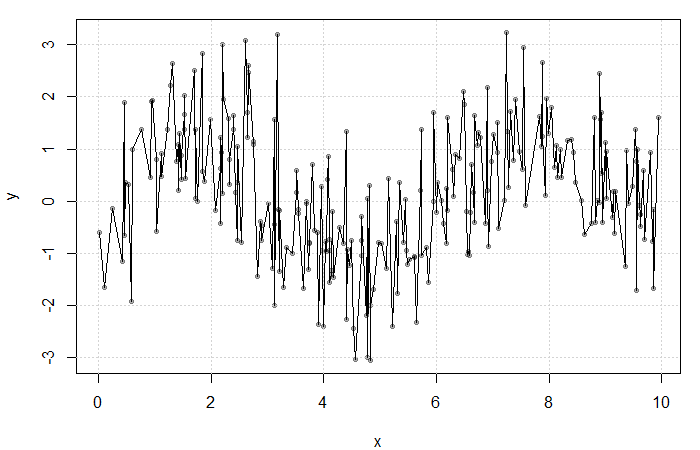Result of averaging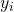at each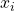. The fit is extremely rough due to gaps inand lowfrequency at each.

Define the neighbourhood aroundas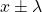for some bandwidth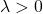. Then, a simple non-parametric estimate of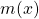can be constructed as average of the‘s corresponding to thewithin this neighbourhood. That is,

(1)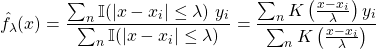where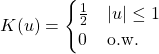is the uniform kernel. This estimator, referred to as the Nadaraya-Watson estimator, can be generalized to any kernel function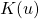(see my previous blog bost). It is, however, convention to use kernel functions of degree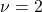(e.g. the Gaussian and Epanechnikov kernels).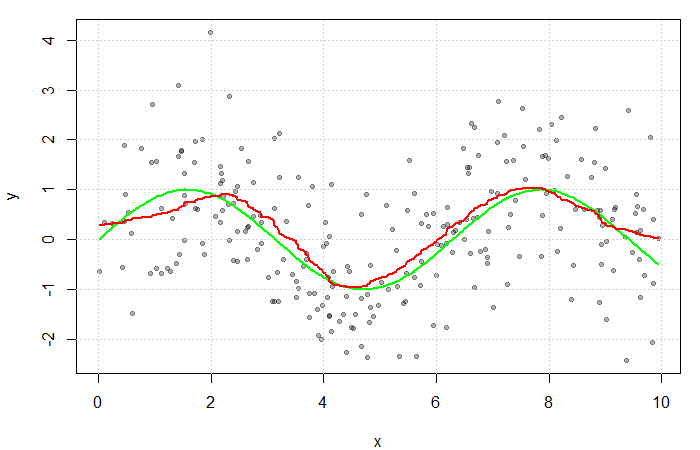The red line is the result of estimatingwith a Gaussian kernel and arbitrarily selected bandwidth of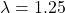. The green line represents the true function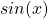.

## Kernel and Bandwidth Selection

The implementation of a kernel estimator requires two choices:

the kernel,, and
the smoothing parameter, or bandwidth,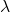.

Kernels are often selected based on their smoothness and compactness. We prefer a compact kernel to ensure that only data local to the point of interest is considered. The optimal choice, under some standard assumptions, is the Epanechnikov kernel. This kernel has the advantages of some smoothness, compactness, and rapid computation.

The choice of bandwidthis critical to the estimator’s performance and far more important than the choice of kernel. If the smoothing parameter is too small, the estimator will be too rough; but if it is too large, we risk smoothing out important function features. In other words, choosinginvolves a significant bias-variance trade-off.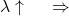smooth curve, low variance, high bias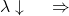rough curve, high variance, low bias

The simplest way of selectingis to plot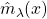for a range of differentand pick the one that looks best. The eye can always visualize additional smoothing, but it is not easy to imagine what a less smooth fit might look like. For this reason, it is recommended that you choose the least smooth fit that does not show any implausible fluctuations.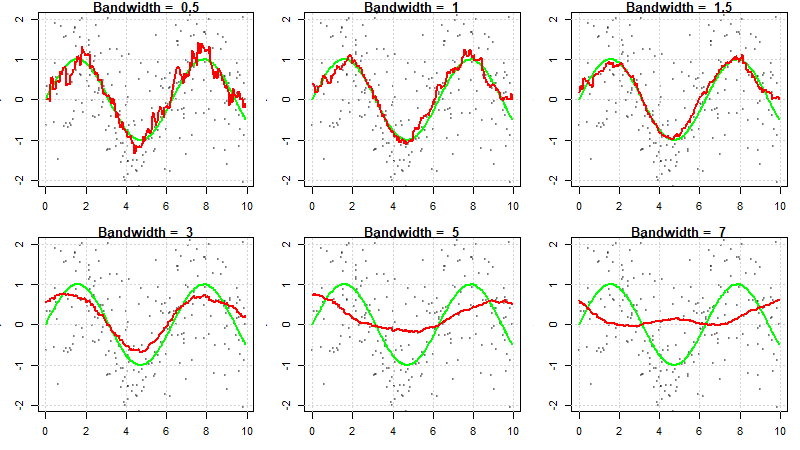Kernel regression fits for various values of.

## Cross-Validation Methods

Selecting the amount of smoothing using subjective methods requires time and effort. Automatic selection ofcan be done via cross-validation. The cross-validation criterion is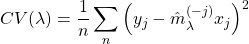where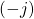indicates that point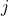is left out of the fit. The basic idea is to leave out observationand estimatebased on the other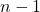observations.is chosen to minimize this criterion.

True cross-validation is computationally expensive, so an approximation known as generalized cross-validation (GCV) is often used. GCV approximates CV and involves only one non-parametric fit for eachvalue (compared to CV which requires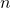fits at each).

In order to approximate CV, it is important to note that kernel smooths are linear. That is,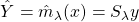where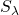is an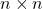smoothing matrix.is analogous to the hat matrix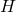in parametric linear models.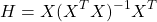It can be shown that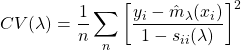where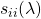is the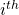diagonal element of(hence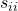is analogous to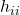, the leverage of theobservation). Using the smoothing matrix,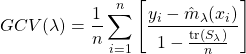where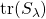is the trace of. In this sense, GCV is analogous to the influence matrix.

Automatic methods such as CV often work well but sometimes produce estimates that are clearly at odds with the amount of smoothing that contextual knowledge would suggest. Therefore, it is essential to exercise caution when using them, and it is recommended that they be used as a starting point.